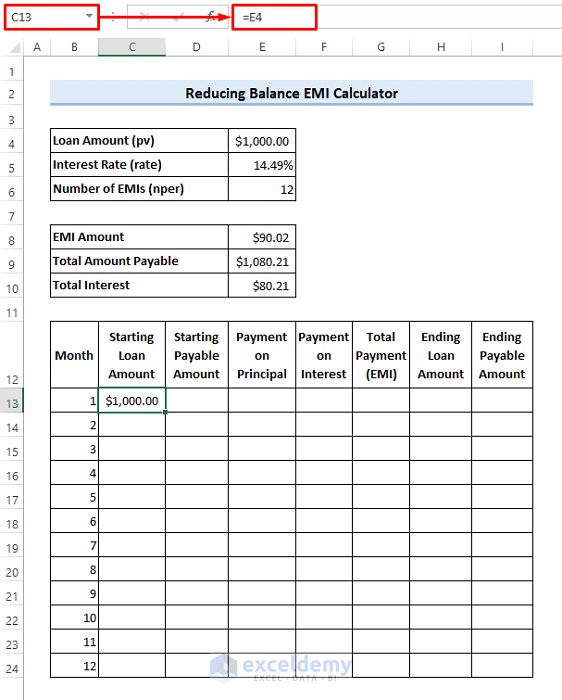# Reducing Balance EMI Calculator in Excel Sheet (Create with Easy Steps)

Get FREE Advanced Excel Exercises with Solutions!

This article illustrates how to create a reducing balance EMI calculator excel sheet. The rate of interest diminishes with time in the case of loans based on reducing balance EMI. Therefore, taking loans based on reducing balance EMI can be a better deal than on fixed balance EMI.

For example, the EMI amount for a \$5000 loan at 9% fixed interest rate for a 12 month period will be = \$5000/12 + \$5000*9%/12 = \$454.17. On the other hand, the EMI amount at the reducing balance interest rate will be \$437.26. Therefore, it can be a better deal to take a loan at the reducing balance interest rate even if the interest rate is a little higher. Follow the article to create a reducing balance EMI calculator in your excel sheet to find out the better deal for yourself.

## Steps to Create a Reducing Balance EMI Calculator in Excel Sheet

Assume you have secured a loan of \$1000 at the annual rate of 14.49%. You can repay the loan in 12 equated monthly installments (EMI).Follow the steps below to create a reducing balance EMI calculator excel sheet using that information.

### 📌 Step 1: Calculate EMI Amount with PMT Function

• First, enter the following formula in cell E8 to estimate the EMI amount. Here the PMT function returns negative numbers. Therefore a negative sign has been used at the beginning of the formula.
`=-PMT(E5/12,E6,E4,0,0)`### 📌 Step 2: Estimate Total Amount Payable

• Then enter the following formula in cell E9 to calculate the total payable amount.
`=E8*E6`### 📌 Step 3: Calculate Total Interest

• Next enter the following formula in cell E10 to get the total interest.
`=E9-E4`### 📌 Step 4: Create a Reducing Balance EMI Table

• Now enter 1 to 12 in cells B13 to B24 respectively. After that, enter the following formula in cell C13.
`=E4`

###• Then, enter the following formula in cell D13.
`=E9`• Next, apply the following formula in cell E13 to calculate the monthly payment need to be made on the principal loan amount. Then drag the fill handle icon down to cell E24. Here the PPMT function also returns negative values as it considers the payment as cash outflow.
`=-PPMT(\$E\$5/12,B13,\$E\$6,\$E\$4,0,0)`• After that, enter the following formula in cell F13 to calculate the monthly payment need to be made on the interest. Then, drag the fill handle icon down to cell F24. Here the IPMT function also considers the payments as cash outflow. So a negative sign is used at the beginning to avoid a negative result.
=-IPMT(\$E\$5/12,B13,\$E\$6,\$E\$4,0,0)• Now enter the following formula in cell G13 to calculate the total monthly payment. You can see that the result is equal to the EMI amount as it must be.
`=SUM(E13:F13)`• Now enter the following formula in cell H13 to calculate the remaining loan balance at the end of the month. Then, drag the fill handle icon down to cell H24.
`=C13-E13`• After that, enter the following formula in cell I13 to calculate the total amount payable at the end of the month. Then, drag the fill handle icon down to cell I24.
`=D13-G13`• Then enter the following formula in cell C14. Next drag the fill handle icon down to cell C24.
`=H13`• Then enter the following formula in cell D14. After that, drag the fill handle icon down to cell D24.
`=I13`### 📌 Step 5: Finalize the EMI Calculator Sheet

• Finally, you will see the following result.• Now enter the following formula in cell E25 to verify the results. Then drag the fill handle icon to cell G25. Then you will see that the total payment made on the loan, the total payment made on interest, and the total amount payable match the results calculated earlier.
`=SUM(E13:E24)`Note:

Don’t forget to use a negative sign before the formulas containing the financial function to avoid any negative results.

## Conclusion

Now you know how to create a reducing balance EMI calculator in an excel sheet. Do you have any further queries or suggestions? Please let us know using the comment section below. You can also visit our ExcelDemy blog to explore more about excel. Stay with us and keep learning.

## Related ArticlesMd. Shamim Reza

Hello there! This is Md. Shamim Reza. Working as an Excel & VBA Content Developer at ExcelDemy. We try to find simple & easy solutions to the problems that Excel users face every day. Our goal is to gather knowledge, find innovative solutions through them and make those solutions available for everybody. Stay with us & keep learning.

We will be happy to hear your thoughtsAdvanced Excel Exercises with Solutions PDF